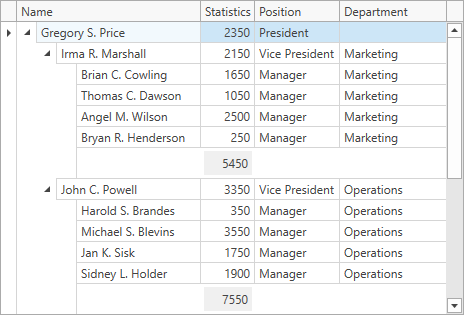# TreeListView.CustomSummary Event

Enables you to calculate summary values manually.

Namespace: DevExpress.Xpf.Grid

Assembly: DevExpress.Xpf.Grid.v22.2.dll

NuGet Package: DevExpress.Wpf.Grid.Core

## Declaration

``public event TreeListCustomSummaryEventHandler CustomSummary``

## Event Data

The CustomSummary event's data class is TreeListCustomSummaryEventArgs. The following properties provide information specific to this event:

Property Description
FieldValue Gets the processed field value.
IsNodeSummary Gets whether the processed row values are used to calculate a node summary.
IsTotalSummary Gets whether the processed row values are used to calculate a total summary.
IsTotalValueReady Gets or sets whether the Calculation stage of the custom summary calculation process should be skipped.
Node Gets the processed node.
SummaryItem Gets a summary item whose value is being calculated.
SummaryProcess Gets a value indicating the calculation stage.
TotalValue Gets or sets the total summary value.

## Remarks

Total summaries contain predefined aggregate functions. These functions allow you to calculate the following:

• The number of data rows (Count)
• The maximum and minimum values (Max and Min)
• The sum and the average value (Sum and Average)

Use the CustomSummary event to apply custom rules to calculate summaries. This event allows you to implement custom aggregate functions or use a custom algorithm to calculate summary values.

If you want to maintain a clean MVVM pattern and specify custom summaries in a View Model, create a command and bind it to the CustomSummaryCommand property.

## Example

The following example demonstrates how to calculate custom node summaries in the TreeListView. To do this, handle TreeListView’s CustomSummary event and use the IsNodeSummary property to determine whether to calculate node summaries.``````<dxg:TreeListControl AutoGenerateColumns="AddNew" Name="grid">
<dxg:TreeListControl.View>
<dxg:TreeListView AutoExpandAllNodes="True" AutoWidth="True"
KeyFieldName="ID" ParentFieldName="ParentID"
ShowNodeFooters="True"
CustomSummary="OnCustomSummary">
<dxg:TreeListView.NodeSummary>
<dxg:TreeListSummaryItem FieldName="Statistics" SummaryType="Custom"/>
</dxg:TreeListView.NodeSummary>
</dxg:TreeListView>
</dxg:TreeListControl.View>
</dxg:TreeListControl>
``````
``````void OnCustomSummary(object sender, TreeListCustomSummaryEventArgs e) {
if(e.IsNodeSummary && e.SummaryItem.FieldName == "Statistics") {
if(e.SummaryProcess == CustomSummaryProcess.Calculate) {
e.TotalValue = Convert.ToDouble(e.TotalValue) + (double)e.FieldValue;
}
}
}
``````

The following code snippet (auto-collected from DevExpress Examples) contains a reference to the CustomSummary event.

Note

The algorithm used to collect these code examples remains a work in progress. Accordingly, the links and snippets below may produce inaccurate results. If you encounter an issue with code examples below, please use the feedback form on this page to report the issue.